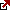This calculator below you can use to calculate the spread cost and as a spread betting leverage calculator:

First, let us explain why the bid-ask spread is a transaction cost. Investors always buy at the ask and sell at the bid, but consistently a bit higher and always sell slightly lower than the actual price. Since asking prices consistently exceed bid prices, investors “lose” this difference. The spread is the transaction cost. Spreads are trading costs and brokers’ profits.

Forex Spread Cost Calculator is a tool designed to help traders quantify the cost associated with the bid-ask spread of a currency pair and how it can influence their yearly performance. The spread, the difference between the bid and ask prices, is a crucial factor in determining transaction costs in forex trading. Here’s how to calculate the impact of spreads on a trading account:

• Let’s call this number T (for example, if you trade five times a day, then T = 5).

• To determine the total trades in a year, multiply the number of trades per day (T) by the number of trading days in a year (usually around 252). Don’t forget, for each trade, you have both an entry and an exit, so multiply the result by 2.
• Let’s call this number L (for instance, if your leverage is 1:100, then L = 100).

b. Account equity:

• This is how much money you have in your trading account. Let’s call this E.

• Let’s call this Sold.

b. New spread value in pips:

• Let’s call this Snew.

4. Yearly Return Impact:a. Yearly past return in percents:
• Let’s say this is R% (for instance, if your return was 10%, then R = 10).

First, calculate your total annual savings due to the reduced spread to find out how the new spread could impact your returns. This is found by multiplying the spread cost per trade by the total number of trades in a year.

Latest posts by Fxigor (see all)

Fxigor

Igor has been a trader since 2007. Currently, Igor works for several prop trading companies. He is an expert in financial niche, long-term trading, and weekly technical levels. The primary field of Igor's research is the application of machine learning in algorithmic trading. Education: Computer Engineering and Ph.D. in machine learning. Igor regularly publishes trading-related videos on the Fxigor Youtube channel. To contact Igor write on: igor@forex.in.rs

Trade gold and silver. Visit the broker's page and start trading high liquidity spot metals - the most traded instruments in the world.